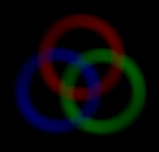Lomont.org

(Also lomonster.com and clomont.com)

Published

A post to test site features such as tables, math, code…

Testing SCSS/SASS text

Code

int main(void)
{
printf("Testing %d\n",10);
}


Math inline $x=\frac{-b\pm\sqrt{b^2-4ac}}{2a}$ is here

And here $$x=\frac{-b\pm\sqrt{b^2-4ac}}{2a}$$ is here

Block: $$x=\frac{-b\pm\sqrt{b^2-4ac}}{2a}$$

Math inline $x=-b$ is here

More block $x=\frac{-b\pm\sqrt{b^2-4ac}}{2a}$

An imageSome tables

tables test
entry 1 entry2

Categories:

Tags: